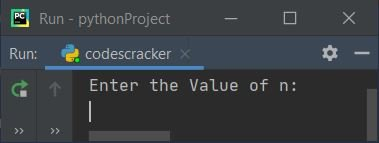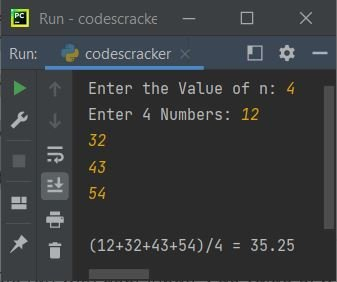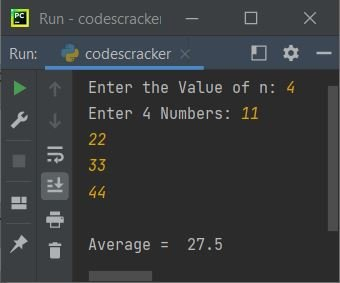# Python Program to Find Average of n Numbers

In this article, we've created some programs in Python, to find and print average of n numbers entered by user at run-time. Here are the list of programs:

• Find Average of n Numbers using for loop
• using while loop
• using function
• using class and object

For example, if the value of n entered by user is 5. And five numbers entered as 10, 20 ,30, 40, 50. Then average of these numbers gets calculated as:

```average = (10+20+30+40+50)/5
= (150/5)
= 30```

## Find Average of n Numbers using for Loop

To find average or arithmetic mean of n numbers entered by user in Python, you have to ask from user to enter the value of n, and then n set of numbers, find and print the average or arithmetic mean value of all those numbers as shown in the program given below:

```print("Enter the Value of n: ")
n = int(input())
print("Enter " +str(n)+ " Numbers: ")
nums = []
for i in range(n):
nums.insert(i, int(input()))

sum = 0
for i in range(n):
sum = sum+nums[i]

avg = sum/n
print("\nAverage = ", avg)```

Here is the initial output produced by this program:Now supply inputs say 5 as value of n and then enter any five numbers say 12, 43, 54, 10, and 23 one by one. Press `ENTER` key find and print the average value of given numbers as shown in the snapshot given below:Note - The str() method converts any type of value to a string type value.

The dry run of following block of code:

```for i in range(n):
nums.insert(i, int(input()))```

goes in this way:

• The loop variable i starts with 0, since there is only one argument is given to range()
• The value to range() is the value of n. Therefore, if 5 is the value of n, then the loop gets executed 5 times with value of i from 0 to 4
• So at first execution of the loop, the statement, nums.insert(i, int(input())) states that, the value entered by user gets inserted at ith index of nums
• That is, first value gets stored in nums
• In similar way, the second, third, fourth, and fifth value gets stored in nums, nums, nums, and nums respectively

#### Modified Version of Previous Program

This program uses end=, that is used to skip an automatic printing of newline using print(). The append() method is also used to insert element to the list. Since, the inserting of element to list starts with very first (zeroth) index, therefore better to use append(), instead of insert()

```print("Enter the Value of n: ", end="")
n = int(input())
print("Enter " +str(n)+ " Numbers: ", end="")
arr = []
for i in range(n):
arr.append(int(input()))

sum = 0
for i in range(n):
sum = sum+arr[i]
if i==0:
print(end="\n(" +str(arr[i]))
elif i==(n-1):
print(end="+" +str(arr[i])+ ")")
else:
print(end="+" +str(arr[i]))

print(end="/" +str(n)+ " = ")
avg = sum/n
print(end=str(avg))```

Here is its sample run with user input, 4 as value of n and 12, 32, 43, 54 as four numbers:## Find Average of n Numbers using while Loop

This program uses while loop to do the same task as of previous program. In this program, the value 0 to i gets initialized to it, before starting the loop. And its value gets incremented from inside its body. Because, while loop only contains the condition checking part.

```print("Enter the Value of n: ", end="")
n = int(input())
print("Enter " +str(n)+ " Numbers: ", end="")
nums = []
i = 0
while i<n:
nums.append(int(input()))
i = i+1

sum = 0
i = 0
while i<n:
sum = sum+nums[i]
i = i+1

avg = sum/n
print("\nAverage = ", avg)```

Here is its sample run with user input, 4 as n's value and 11, 22, 33, 44 as four numbers:## Find Average of n Numbers using Function

This program does the same job using a user-defined function named avg(). This function takes two arguments. The first argument refers to the list (that contains n set of numbers). And the second argument refers to the size of list (value of n). It returns the average value.

```def avg(arr, tot):
sum = 0
for i in range(n):
sum = sum+arr[i]
avg = sum/tot
return avg

print("Enter the Value of n: ", end="")
n = int(input())
print("Enter " +str(n)+ " Numbers: ", end="")
nums = []
for i in range(n):
nums.append(int(input()))

res = avg(nums, n)
print("\nAverage = ", res)```

This program produces the same output as of previous program.

## Find Average of n Numbers using Class

This is the last program of this article, created using class and object, an object-oriented feature of Python.

```class CodesCracker:
def avg(self, arr, tot):
sum = 0
for i in range(n):
sum = sum+arr[i]
avg = sum/tot
return avg

print("Enter the Value of n: ", end="")
n = int(input())
print("Enter " +str(n)+ " Numbers: ", end="")
nums = []
for i in range(n):
nums.append(int(input()))

obj = CodesCracker()
res = obj.avg(nums, n)

print("\nAverage = ", res)```

From above program, the following statement:

`obj = CodesCracker()`

is used to assign all the properties of class CodesCracker to the object obj. So that, this object can be used to access the member function (avg()) of the same class using dot (.) operator. Rest of the things works similar to a normal function.

#### Same Program in Other Languages

Python Online Test

« Previous Program Next Program »